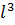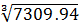### Sample Problem

A recent study found that the weight of a certain species of fish living in the Bahamas can be modeled by the function w = 0.0342, where w is measured in grams and, the length of the fish, is measured in centimeters. Calculate the approximate length of a fish that weighs 250 grams. Round your answer to the nearest tenth of a centimeter.

l = cm

#### Solution

w = 0.0342250 = 0.03427309.94 =l =≈19.4